SNVS480J January   2007  – July 2020

PRODUCTION DATA.

1. Features
2. Applications
3. Description
1.     Device Images
4. Revision History
5. Pin Configuration and Functions
6. Specifications
7. Detailed Description
1. 7.1 Overview
2. 7.2 Functional Block Diagram
3. 7.3 Feature Description
4. 7.4 Device Functional Modes
8. Application and Implementation
1. 8.1 Application Information
2. 8.2 Typical Application
1. 8.2.1 Design Requirements
2. 8.2.2 Detailed Design Procedure
3. 8.2.3 Application Curves
9. Power Supply Recommendations
10. 10Layout
1. 10.1 Layout Guidelines
2. 10.2 Layout Examples
11. 11Device and Documentation Support
12. 12Mechanical, Packaging, and Orderable Information

• DGS|10

#### 8.2.2.9 RSNS, RS2, and Current Limit

The current sensing resistor, RSNS, is used for steady-state regulation of the inductor current and to sense overcurrent conditions. The slope compensation resistor is used to ensure control loop stability, and both resistors affect the current limit threshold. The RSNS value selected must be low enough to keep the power dissipation to a minimum, yet high enough to provide good signal-to-noise ratio for the current sensing circuitry. RSNS and RS2 must be set so that the current limit comparator, with a threshold of 0.5 V, trips before the sensed current exceeds the peak current rating of the inductor, without limiting the output power in steady state.

For this example, the peak current at VIN(MIN) is 2.5 A, while the inductor itself is rated to 3.2 A. The threshold for current limit, ILIM, is set slightly between these two values to account for tolerance of the circuit components, at a level of 3 A. The required resistor calculation must take both the switch current through RSNS and the compensation ramp current flowing through the internal 2-kΩ RS1 and RS2 resistors into account. RSNS must be selected first because it is a power resistor with more limited selection. Equation 36 and Equation 37 must be evaluated at VIN(MIN) when duty cycle is highest.

Equation 36.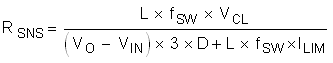Equation 37.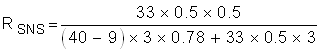where

• L is in µH
• fSW in MHz

The closest 5% value is 100 mΩ. Power dissipation in RSNS can be estimated by calculating the average current. The worst-case average current through RSNS occurs at minimum input voltage/maximum duty cycle and can be calculated with Equation 38 and Equation 39.

Equation 38.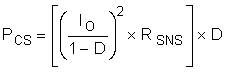Equation 39. PCS = [(0.5 / 0.22)2 × 0.1] × 0.78 = 0.4 W

For this example, a 0.1 Ω ±1%, thick-film chip resistor in a 1210 case size rated to 0.5 W is used.

With RSNS selected, RS2 can be determined using Equation 40 and Equation 41.

Equation 40.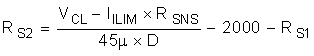Equation 41.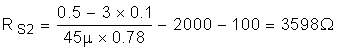The closest 1% tolerance value is 3.57 kΩ.